# Rees semi-group of matrix type

Jump to: navigation, search

A semi-group theoretical construction defined as follows. Letbe an arbitrary semi-group, letand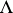be (index) sets and let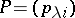be a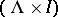-matrix over, i.e. a mapping from the Cartesian product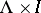into. The following formula defines an operation on the set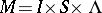: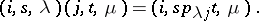Thenis a semi-group, called a Rees semi-group of matrix type overand denoted by; the matrixis called the sandwich matrix of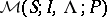. Ifis a semi-group with zero 0, then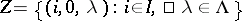is an ideal in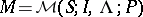and the Rees quotient semi-group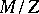(see Semi-group) is denoted by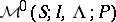; in the case whenis a group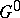with an adjoined zero, instead ofone writes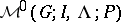and calls it a Rees semi-group of matrix type over the groupwith an adjoined zero. The groupis called the structure group for the semi-groupsand.

Another representation of the Rees semi-group of matrix type over a semi-groupwith zero and-sandwich matrixis realized in the following way. An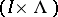-matrix overis called a Rees matrix if it does not contain more than one non-zero element. Let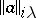be the Rees matrix overthat hasin the-th row and-th column, and zeros in all other places. On the set of all-Rees matrices overone can define an operation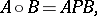(1)

where on the right-hand side is the "ordinary" matrix product. This set becomes a semi-group with respect to this operation. The mapping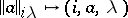is an isomorphism between this semi-group and the semi-group; the notationis used for both of these semi-groups. Formula (1) provides an explanation of the term "sandwich matrix" for. Ifis a group, then the semi-groupis regular if and only if each row and each column of the matrixcontains a non-zero element; any semi-group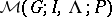is completely simple (cf. Completely-simple semi-group), any regular semi-groupis completely-simple. The converse of the last two statements gives the main content of Rees's theorem : Any completely-simple (completely-simple) semi-group can be isomorphically represented as a Rees semi-group of matrix type over a group (as a regular Rees semi-group of matrix type over a group with an adjoined zero). Ifandare isomorphic, then the groupsand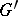are isomorphic,andhave the same cardinality, andandhave the same cardinality. Necessary and sufficient conditions for isomorphy of the semi-groupsand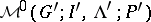are known, and together with the just-mentioned conditions they include a quite definite relation between the sandwich matricesand(see ). In particular, any completely-simple semi-group can be isomorphically represented as a Rees semi-group of matrix type in whose sandwich matrixeach element in a given row and a given column is either 0 or the identity element of the structure group; such a sandwich matrix is called normalized. Similar properties are valid for completely-simple semi-groups.